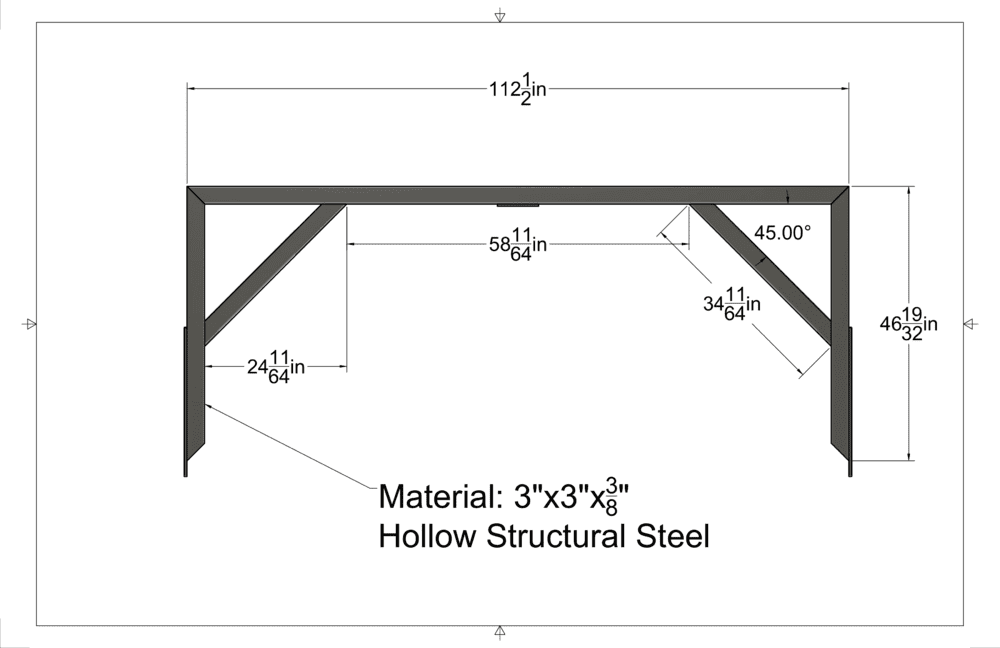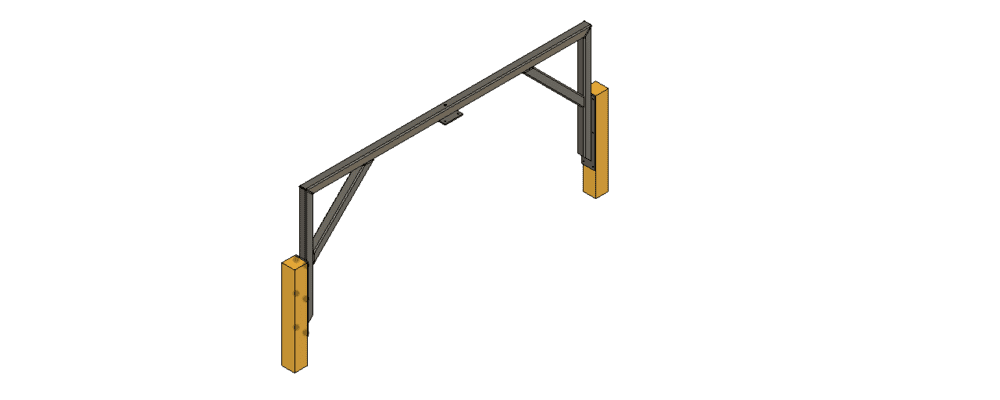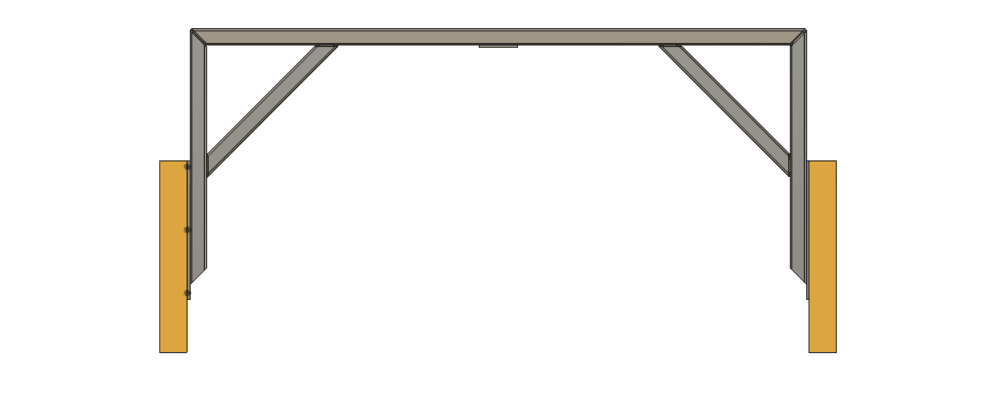# Need a Hand Calculating the Maximum Load on a Structure

Summary:
Calculating Maximum Load of Theoretical Model
Basically looking to find out what the maximum amount of load you could put on the center of a structure like this would be, in theory.

Top Beam:
The top "Beam" of the assembly in the drawing is 3"x 3"x 3/8" square tubing, 112.5" long

Supporting Beams:
The beams supporting each end of the Top Beam are the same 3"x 3"x 3/8" Tubing 46.59375" long, mitered at a 45 degree angle and theoretically welded or bolted

45 Degree Braces:
There are also two 45 degree braces to add some support to the center of the Top Beam. These are 34.171" long tip to tip, they are 45 degree mitered on each end and theoretically welded or boltedBasically I've crunched numbers with some equations I found online for applying load to the center of a hollow square tube, but without just plugging in different amounts of force I'm unsure of how to figure out what the maximum load you could apply would be. Not really sure how to calculate in for the angled supports or even figure out what the Top Beam can actually take for load before plastic deformation occurs. My math comes out as follows:

Calculating the Moment Of Inertia

(Height^3 x Width)/12-(Inside Height^3 x Inside Width)/12
So
(3^3 x 3)/12-(2.25^3 x 2.25)/12
(81)/12-(25.62890625)/12
MoI = 4.614257812in^4

ASTM 1085 Spec Says HSS MoI = 3.89in^4

Then for Calculating Deflection, Assuming the Modulus of Elasticity is 29,000,000 for Steel

(Length^3 x Force Applied/(3 x MoE x MoI))
So
(112.5^3 x 10,000lb/(3 x 29,000,000 x 4.614257812))
(112.5^3 x 10,000lb/(401440429.644))
( 14238281250)(401440429.644)
Deflection = 35.4679802956" (With Calculated MoI)

Deflection = 42.07156945" (With ASTM 1085 MoI)

Obviously this is a lot of deflection in the Top Beam with the numbers I have plugged in for load but I know that I'm also not figuring in for the 45 degree braces. I did do some calculations for deflection using 88.328125" as the Top Beam length (which came out to a deflection of 17.16626947", about half of what I got with the full length), basically I figured that if I treated the math as if the Top Beam's endpoints were in the center of the space where the 45 degree braces intersect the Top Beam it would get me close to the actual deflection but I know that that's certainly not an accurate way to do that. The other thing is if I do the math treating where the 45 degree braces intersect the Top Beam as the end points of the Top Beam, and plug in a 1500lb load I get a deflection of 0.7480622661", and I have a really hard time believing that a 58.5" long section of 3"x 3"x 3/8" tubing would deflect more than 1/16th of an inch with only 1500lb load on it. If someone could walk me through figuring out how much load this design can actually take that would be amazing!

Last edited:

## Answers and Replies

JBA
Science Advisor
Gold Member
For the case where you are calculating the deflection of the 58.5" length section of the the top beam between the two braces; for a center single load, if you assume that each end of the top beam is simply supported, the correct formula for the max deflection at the center load point of the beam is: d = W * L^3 / (48 *E * I). If you assume both ends are rigidly restrained, the formula is: d = W * L^3 / (192 * E * I).

For your actual case the situation is a bit more complex; because, if the two end supports are bolted at their lower ends as indicated in your diagram, then those supports will be subjected to a bending moment from the top beam connections bending them inward and this will effect the stress and deflection of the top beam.

jrmichler
Mentor
A problem like this is tackled in steps.

Step 1: Calculate the top beam as 112.5" long and simply supported. This is a conservative calculation, so if the stress and deflection are acceptable, you are finished.

Step 2: Calculate as a frame with two verticals and a horizontal member. Ignore the angle braces, and assume simple supports at the floor. Roark's Formulas for Stress and Strain has the equations for both concentrated and distributed loads for this case. This is a conservative calculation, so if the stress and deflection are acceptable, you are finished.

Step 3: If the frame is solidly fastened to a thick concrete floor, such that the floor connections stay rock solid under load, then it can be calculated as a frame with fixed end supports. Roark has the equation for both concentrated and distributed loads for this case without the angle braces. This calculation will only be conservative if the floor is significantly more rigid than the frame. The angle braces may or may not make a contribution to the strength and stiffness of the frame, depending on exactly where they connect to the vertical members, so they are ignored for this hand calculation.

Step 4: If the results from Step 3 show that it is almost strong/stiff enough, then you need FEA to calculate the strength and stiffness of the entire structure, including the angle braces. A simple FEA analysis will look at the two cases of simple and fixed end supports at the floor. A better FEA analysis will include the connection to the floor, and the effect of the floor.

Hint: Always use published values for MOI of standard steel sections. The published values take into account the rounded corners.

If the horizontal is bolted to the verticals, then it can only be analyzed as indicated above if the bolted connection is a moment connection.

•berkeman
Would it be a good idea to average the answers for the Rigid Edge Deflection and Simply Supported Deflection formulas together to aim in the middle of both values? Above mentioned structure is mounted to 5" wide posts that go up and down via a welded on 1/2" plate with six 1/2-13" Grade 8 bolts hold it on. The Top Beam is modeled as being welded on that 45 degree miter cut to the Supporting Beams, and the 45 Degree Braces are welded in place.If you share the 3D model I can perform Finite Element Analysis of this structure for you. Such simulation should give you the most accurate results but it’s still good to verify it with hand calculations as described by @jrmichler.

Last edited by a moderator:
256bits
Gold Member
Summary: Calculating Maximum Load of Theoretical Model

Tubing 46.59375
Just a comment about

That's 46 - 19/32 inch ( exactly )
Do you need that much precision for your structure?
Your tube cutter, welder or bolt hole driller will be asking for a tolerance of the dimensions you have laid out.
And they usually charge more for higher tolerances.

Deflection = 35.4679802956
[/QUOTE]
Would anyone notice a billionth of an inch either way?
( 15/32 is 0.46875 inch )

And
for the angle braces
Either two of 24 - 11/64, 34 - 11/64, and 45 degrees, are correct, but not all three.
One is a reference for the fabricator, so he doesn't get frustrated.
Both braces may not turn out to be symmetric, if that is what is to be desired.
Actually thinking about it, the 58 - 11/64 could be a ref, the way the dimensions are laid out.